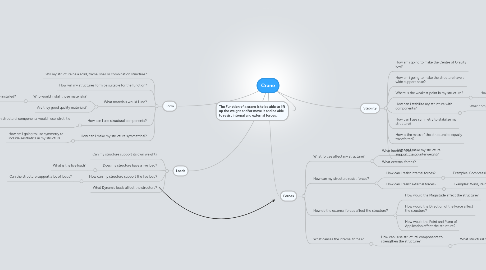# Crane

Get Started. It's FreeCrane## 1. Stability

### 1.3. Where is the weakest point in my structure?

1.3.1. How am I going to make that part strong?

### 1.4. How can i stabilize my structure with components?

1.4.1. What components would I use?

## 2. Form

### 2.3. What materials would I use?

2.3.1. Who would install those materials?

2.3.1.1. How would they be installed?

2.3.2. Are they good quality materials?

### 2.4. How can I use structural components?

2.4.1. Which structural components would I use, strut, tie etc.?

2.4.1.1. How would it help my structure?

### 2.5. How can i make my structure symmetrical?

2.5.1. How am I going to use symmetry to include aesthetics in my structure?

### 3.2. Does my structure have a live load?

3.2.1. What is the live load?

### 3.3. How can my structure support the live load?

3.3.1. Can the structure support a lot of loads?

## 5. Forces

### 5.1. What forces affect my structure?

5.1.1. What internal forces?

5.1.2. What external forces?

### 5.2. How can my structure resist forces?

5.2.1. How can I resist internal forces?

5.2.1.1. Examples: Compression, torsion, shear, tension

5.2.2. How can I resist external forces?

5.2.2.1. Examples: Wind, Rain etc.

### 5.3. How do the external forces affect the structure?

5.3.1. How would the Magnitude affect the structure?

5.3.2. How would the Direction of the Force affect the structure?

5.3.3. How would the Point and Plane of Application affect the structure?

### 5.4. What causes the internal forces?

5.4.1. How can I use structural components to strengthen the structure?

5.4.1.1. What structural components should I use?